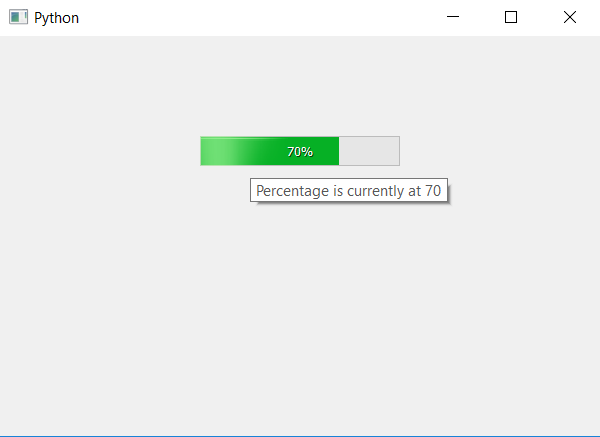GeeksforGeeks App
Open AppBrowser
Continue

# PyQt5 – Progress Bar tool tip

In this article we will see how to set tool tip to a progress bar. Tool tip is basically tip showed up on screen when cursor hover upon the progress bar. Tool tip will basically used to tell the user about the percentage or how much time is left to complete, in order to set the tool tip we use `setToolTip` method.

Syntax : bar.setToolTip(text)

Argument : It takes string as argument.

Action performed : It will set tool tip to progress bar.

Below is the implementation.

 `# importing libraries``from` `PyQt5.QtWidgets ``import` `*` `from` `PyQt5 ``import` `QtCore, QtGui``from` `PyQt5.QtGui ``import` `*` `from` `PyQt5.QtCore ``import` `*` `import` `sys`` ` ` ` `class` `Window(QMainWindow):`` ` `    ``def` `__init__(``self``):``        ``super``().__init__()`` ` `        ``# setting title``        ``self``.setWindowTitle(``"Python "``)`` ` `        ``# setting background color to window``        ``# self.setStyleSheet("background-color : yellow")`` ` `        ``# setting geometry``        ``self``.setGeometry(``100``, ``100``, ``600``, ``400``)`` ` `        ``# calling method``        ``self``.UiComponents()`` ` `        ``# showing all the widgets``        ``self``.show()`` ` `    ``# method for widgets``    ``def` `UiComponents(``self``):``        ``# creating progress bar``        ``bar ``=` `QProgressBar(``self``)`` ` `        ``# setting geometry to progress bar``        ``bar.setGeometry(``200``, ``100``, ``200``, ``30``)`` ` `        ``# setting the value``        ``value ``=` `70``        ``bar.setValue(value)`` ` `        ``# setting alignment to center``        ``bar.setAlignment(Qt.AlignCenter)`` ` `        ``# setting tool tip``        ``bar.setToolTip(``"Percentage is currently at "` `+` `str``(value))`` ` ` ` ` ` `App ``=` `QApplication(sys.argv)`` ` `# create the instance of our Window``window ``=` `Window()`` ` `# start the app``sys.exit(App.``exec``())`

Output :My Personal Notes arrow_drop_up